### IMO Shortlist 1983 problem 23

Kvaliteta:
Avg: 0,0
Težina:
Avg: 0,0
Let$A$ be one of the two distinct points of intersection of two unequal coplanar circles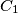$C_1$ and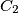$C_2$ with centers$O_1$ and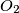$O_2$ respectively. One of the common tangents to the circles touches$C_1$ at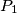$P_1$ and$C_2$ at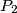$P_2$, while the other touches$C_1$ at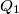$Q_1$ and$C_2$ at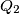$Q_2$. Let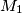$M_1$ be the midpoint of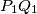$P_1Q_1$ and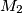$M_2$ the midpoint of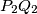$P_2Q_2$. Prove that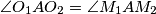$\angle O_1AO_2=\angle M_1AM_2$.
Izvor: Međunarodna matematička olimpijada, shortlist 1983